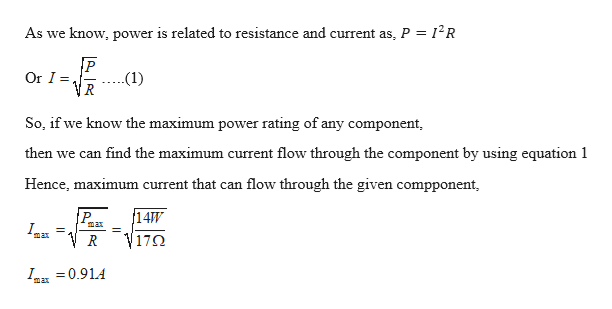# A component with a 17 ohm resistor is rated for use at power levels not exceeding 14 W. How do you determine how much current can safely flow through the component?

Question
8 views

A component with a 17 ohm resistor is rated for use at power levels not exceeding 14 W. How do you determine how much current can safely flow through the component?

check_circle

Step 1

Given:

Resistance of the component = 17 ohm

Maximum Power rating = 14 W

Step 2

Calculating the amount of maximum current th...help_outlineImage TranscriptioncloseAs we know, power is related to resistance and current as P 12R P VR Or I (1) So, if we know the maximum power rating of any component, then we can find the maximum current flow through the component by using equation 1 Hence, maximum current that can flow through the given compponent, 14W P max max 17Ω R I =0.91.4 max fullscreen

### Want to see the full answer?

See Solution

#### Want to see this answer and more?

Solutions are written by subject experts who are available 24/7. Questions are typically answered within 1 hour.*

See Solution
*Response times may vary by subject and question.
Tagged in
SciencePhysics

### Current Electricity